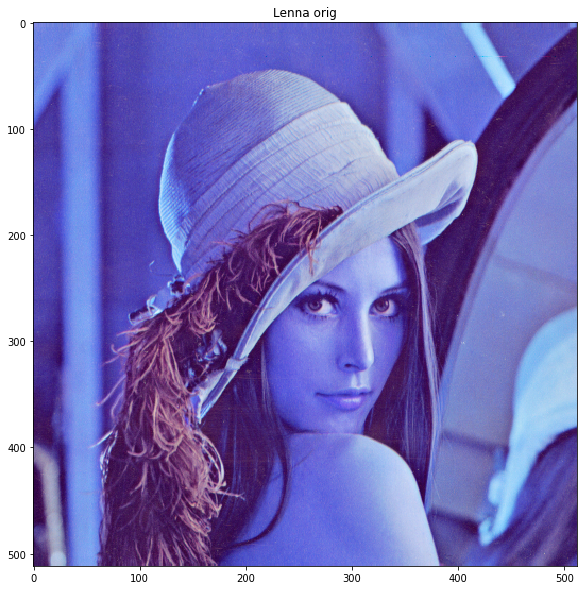## Python Workshop: OpenCVBased on:

http://www.dmi.unict.it/~furnari/teaching/CV1617/lab0/

## Introduction

OpenCV (Open Source Computer Vision Library) is an open source computer vision and machine learning software library. OpenCV was built to provide a common infrastructure for computer vision applications and to accelerate the use of machine perception in the commercial products. [https://opencv.org/about/]## Basic usage

In :
import sys
import matplotlib.pyplot as plt
import cv2  # opencv for python package

figsize = (10, 10)

In :
# to run in google colab
import subprocess
subprocess.call('apt-get install subversion'.split())
subprocess.call('svn export https://github.com/YoniChechik/AI_is_Math/trunk/c_01_intro_to_CV_and_Python/Lenna.png'.split())
subprocess.call(
'svn export https://github.com/YoniChechik/AI_is_Math/trunk/c_01_intro_to_CV_and_Python/opencv_logo.png'.split())


This is how to read and plot an image with opencv

In :
img = cv2.imread("Lenna.png")

In :
plt.figure(figsize=figsize)
plt.imshow(img)
plt.title("Lenna orig")

Out:
Text(0.5, 1.0, 'Lenna orig')We got a weird image colors... This is because OpenCV uses image reading convention of BGR and matplotlib uses RGB.

The fix is easy:

In :
img = cv2.cvtColor(img, cv2.COLOR_BGR2RGB)
plt.figure(figsize=figsize)
plt.imshow(img)
plt.title("Lenna RGB")

Out:
Text(0.5, 1.0, 'Lenna RGB')